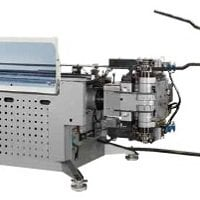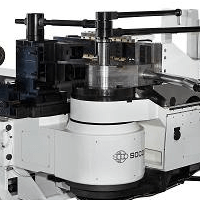# Tube Bending Calculator

July 12, 2022 by
Mike Thomas

If you've ever had to perform the calculations necessary to properly bend tube and pipe you know that while they are a vital part of the bending process, there is often a lot of information to juggle.

In particular, calculating the section area moment of inertia properties of common shapes for tube bending can be time-consuming and complicated.

## What is the section modulus?Typically, structural engineers designing â€œIâ€ beams or T beams calculate section modulus of the cross-sectional area of the beam to understand if the beam will support a particular load or repeated cyclic loading. This is so that building, bridges, and rail road tracks do not fail and have safety factors.

However, section modulus can also relate to bending tubes. When bending tube or pipe, the bender must have enough bend arm toque to overcome a material's section modulus to make a bend into the tube that is permanent.  Also, the material composition determines the deforming of the tubing material past the given material's modulus of elasticity and into the yield strength of the material.

Determining the section modulus of the piece of tube or pipe you need to bend is important because it will allow you to know before you bend if your equipment can successfully complete the bend.

This is important because knowing if a bend will work before you perform it increases your efficiency, saves time, and saves material. Performing a bend incorrectly can lead to tube bulging, tube collapse, and other tube problems. Much better to avoid these problems altogether by taking a bit more time planning your bend and performing calculations.

## What happens if you are not bending round tubes?

How do you know what bender will bend your square, rectangle, non-round shape?  You need to calculate the section area moment of inertia for the round material and compare it to your non-round material.  As long as the round material number is higher than the non-round material, then that particular bender will be capable of bending your part shape.

## Where can you find help with these calculations?

There are a lot of calculations and variables involved in tube bending. We've created a tube bending calculator and tube bending formulas guide to help you navigate all of the calculations, variables, and prep work.

The tube bending section modulus calculator allows you to input the tube specifications into an easy to use calculator. It then calculates the section area moment of inertia properties of common shapes. Some of the most common shapes covered by the calculator are:

•Square
• Round
• Half rounds
• Triangles
• Rectangles
• Trapezoids
• Hexagons
• Octagons

## What is in the Tube Bending Formulas Guide?

The Tube Bending Formulas Guide that is bundled with the calculator covers some of the most common tube bending formulas, including tube inside diameter, wall factor, mandrel nose, radius, and clamp length.